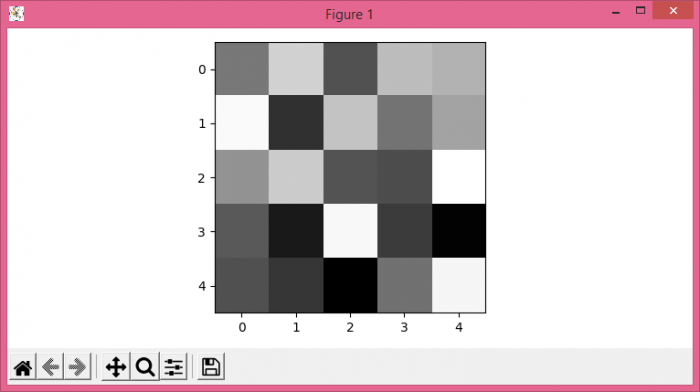# How to save an array as a grayscale image with Matplotlib/Numpy?

To save an array as a grayscale image with Matplotlib/numpy, we can take the following steps −

• Set the figure size and adjust the padding between and around the subplots.
• Create random data with 5☓5 dimension.
• Set the colormap to "gray".
• Plot the data using imshow() method.
• To display the figure, use show() method.

## Example

import numpy as np
from matplotlib import pyplot as plt

plt.rcParams["figure.figsize"] = [7.50, 3.50]
plt.rcParams["figure.autolayout"] = True

arr = np.random.rand(5, 5)
plt.gray()
plt.imshow(arr)

plt.show()

## Output